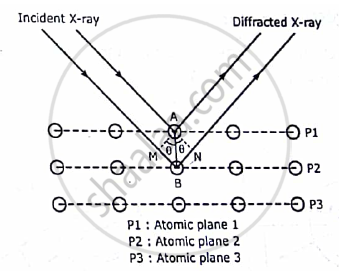# Explain analysis of crystal structure using Bragg’s X ray spectrometer - Applied Physics 1

Short Note

Explain analysis of crystal structure using Bragg’s X ray spectrometer

#### Solution

W.L Bragg’s explained the phenomenon of X-ray diffraction from a single crystal shown
as follows

When a beam of X-rays is incident on a crystal it is scattered by individual atoms of the rich atomic planes. Thus, each atom become a source of scattered radiation. The atomic planes responsible for the X-ray diffraction are called BRAGG’S PLANES. Therefore the sets of Braggs planes constitute the crystal grating. Bragg’s scattering or Bragg’s diffraction is also referred as Braggs reflection. Bragg derived a law called Bragg’s law to explain the X-ray diffraction effect.

Here a beam of X-ray is incident on a set of parallel planes of a crystal. The rays makes a
glancing angle θ and are practically reflected from different successive planes. The phase
relationship of the scattered rays can be determined from their path differences. Here two
parallel X-rays are reflected from two consecutive planes PI and P2. The path differences between then as shownδ=MB+BN=2MB=2AB sinθ
Here AB = d,the interplanar spacing of the crystal.Hence,
δ=2dsinθ
The two diffracted rays reinforce each other when they interfere constructively when their path difference δ is equal to nλ
Hence,            2dsinθ=nλ
This is  called Bragg’s law .

Concept: X-ray Diffraction
Is there an error in this question or solution?